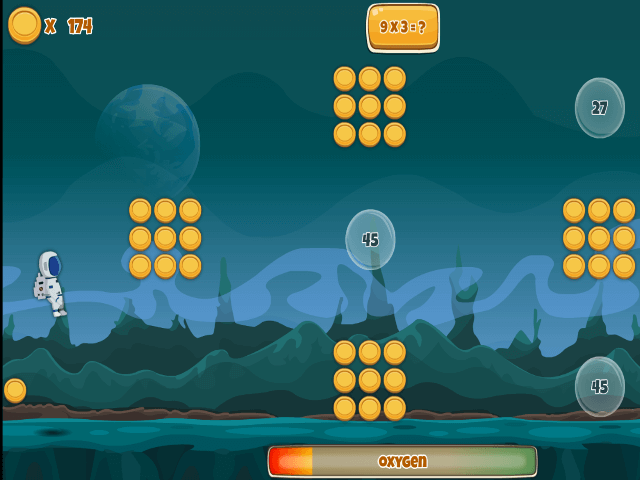## Jetpack Mathematics - Multiplication

From 1st January 2020 this game will only be available to subscribers. A single-user games licence costs only £10 per year. You can register here.

Use the mouse button on a computer or tap on a tablet to guide the spaceman to the correct answers.

 1. Recall and use doubles of all numbers to 10 (Y1) 6. 3 times table (Y3) 11. 6 times table (Y4) 16. Double 3 digit numbers (Y4) 2. 2 times table (Y2) 7. 4 times table (Y3) 12. 7 times table (Y4) 17. Double decimals (1dp) (Y4) 3. 5 times table (Y2) 8. 8 times table (Y3) 13. 9 times table (Y4) 18. Recognise square numbers (up to 15²) (Y5) 4. 10 times table (Y2) 9. Double numbers up to 100 (Y3) 14. 11 times table (Y4) 19. Recognise cube numbers (up to 10³) (Y5) 5. Derive and use doubles of simple two-digit numbers (Y2) 10. Doubles of multiples of 50 up to 500 (Y3) 15. 12 times table (Y4) 20. Double decimals (2dp) (Y5)